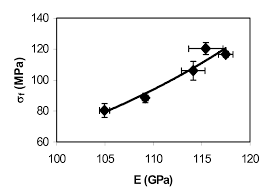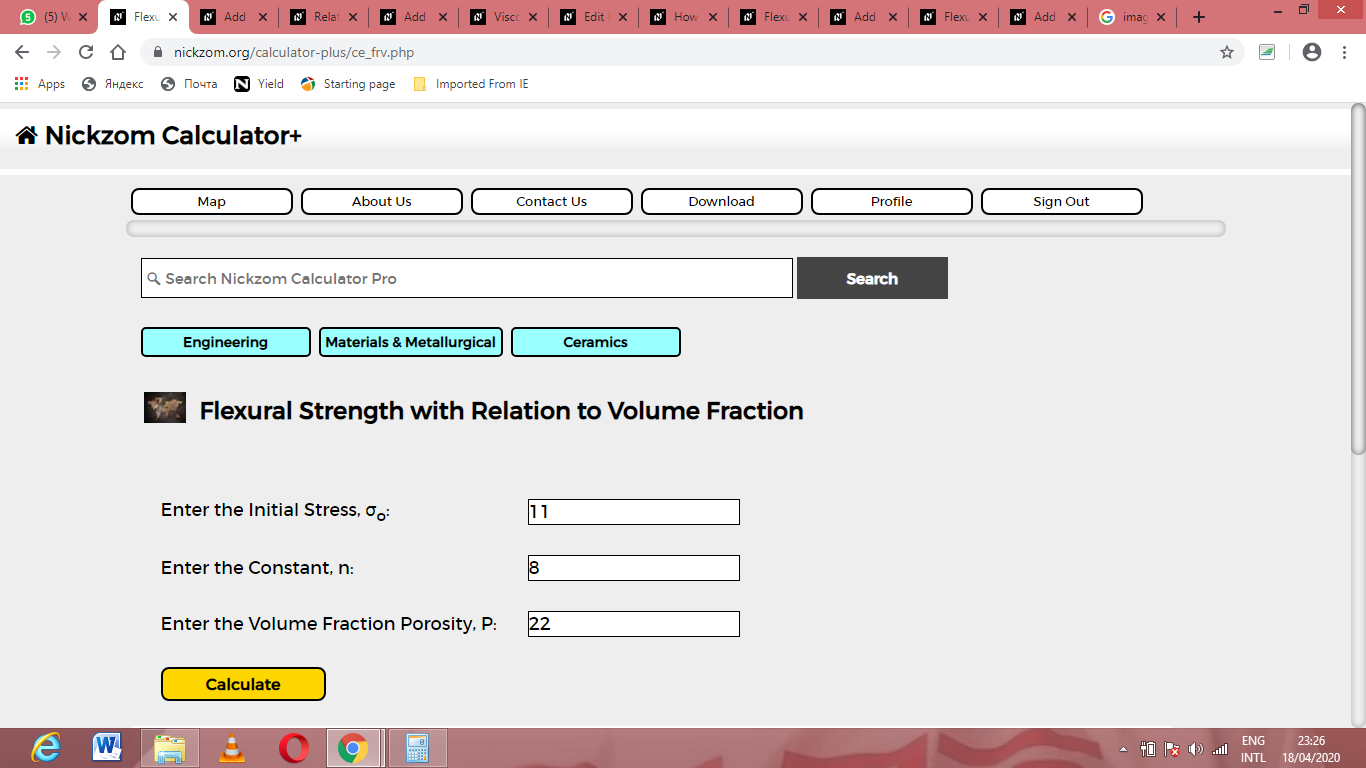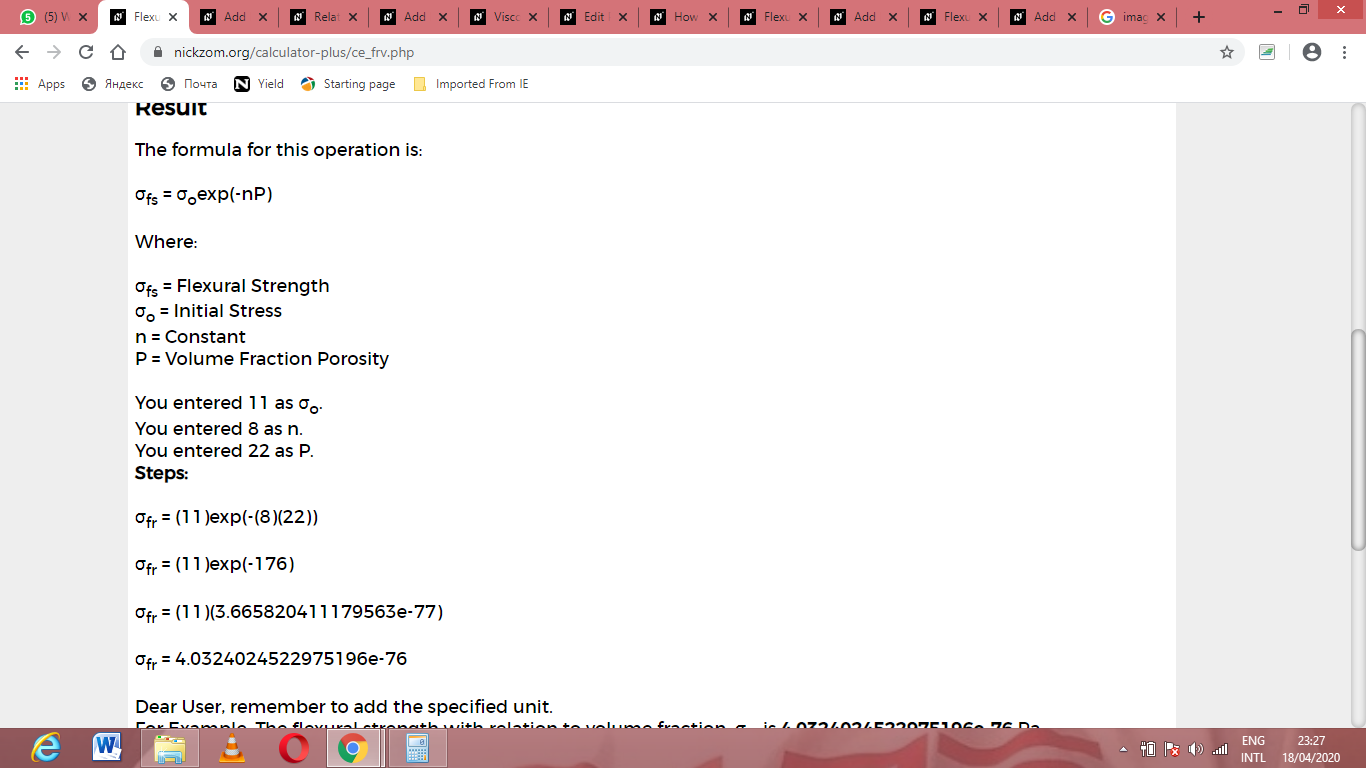# How to Calculate and Solve for Flexural Strength with Relation to Volume Fraction | CeramicsThe image above represents Flexural strength with relation to volume.

To compute for flexural strength with relation to volume, three essential parameters are needed and these parameters are Initial Stress (σo), Constant (n) and Volume fraction porosity (P).

The formula for calculating flexural strength with relation to volume:

σfs = σo exp (-nP)

Where:

σfs = Flexural Strength
σo = Initial Stress
n = Constant
P = Volume Fraction Porosity

Let’s solve an example;
Find the flexural strength when the initial stress is 11, the constant is 8 and the volume fraction porosity is 22.

This implies that;

σo = Initial Stress = 11
n = Constant = 8
P = Volume Fraction Porosity = 22

σfs = σo exp (-nP)
σfr = (11)exp(-(8)(22))
σfr = (11)exp(-176)
σfr = (11)(3.665e-77)
σfr = 4.032e-76

Therefore, the flexural strength is 4.032e-76 Pa.

Calculating the Initial Stress when the Flexural Strength, the Constant and the Volume Fraction Porosity is Given.

σo = σfr / exp (-nP)

Where;

σo = Initial Stress
σfs = Flexural Strength
n = Constant
P = Volume Fraction Porosity

Let’s solve an example;
Find the initial stress when the flexural strength is 20, the constant is 10 and the volume fraction porosity is 8.

This implies that;

σfs = Flexural Strength = 20
n = Constant = 10
P = Volume Fraction Porosity = 8

σo = σfr / exp (-nP)
σo = 20 / exp (-10 x 8)
σo = 20 / exp (-80)
σo = 20 / – 5.54e+34
σo = – 3.61e-34

Therefore, the initial stress is 3.61e-34.

Calculating the Constant when the Flexural Strength, the Initial Stress and the Volume Fraction Porosity is Given.

n = – (In σfr / σo) x 1 / P

Where;

n = Constant
σfs = Flexural Strength
σo = Initial Stress
P = Volume Fraction Porosity

Let’s solve an example;
Find the constant when the flexural strength is 30, the initial stress is 10 and volume fraction porosity is 20.

This implies that;

σfs = Flexural Strength = 30
σo = Initial Stress = 10
P = Volume Fraction Porosity = 20

n = – (In σfr / σo) x 1 / P
n = – (In 30 / 10) x 1 / 20
n = – (In 3) x 0.05
n = – 1.098 x 0.05
n = – 0.0549

Therefore, the constant is – 0.0549.

Calculating the Volume Fraction Porosity when the Flexural Strength, the Initial Stress and Constant is Given.

P = – (In σfr / σo) x 1 / n

Where;

P = Volume Fraction Porosity
σfs = Flexural Strength
σo = Initial Stress
n = Constant

Let’s solve an example;
Find the volume fraction porosity when the flexural strength is 40, the initial stress is 2 and the constant is 12.

This implies that;

σfs = Flexural Strength = 40
σo = Initial Stress = 2
n = Constant = 12

P = – (In σfr / σo) x 1 / n
P = – (In 40 / 2) x 1 / 12
P = – (In 20) x 0.05
P = – 2.99 x 0.05
P = – 0.1495

Therefore, the volume fraction porosity is – 0.1495.

Nickzom Calculator – The Calculator Encyclopedia is capable of calculating the flexural strength.

To get the answer and workings of the flexural strength using the Nickzom Calculator – The Calculator Encyclopedia. First, you need to obtain the app.

You can get this app via any of these means:

To get access to the professional version via web, you need to register and subscribe for NGN 1,500 per annum to have utter access to all functionalities.
You can also try the demo version via https://www.nickzom.org/calculator

Apple (Paid) – https://itunes.apple.com/us/app/nickzom-calculator/id1331162702?mt=8
Once, you have obtained the calculator encyclopedia app, proceed to the Calculator Map, then click on Materials & Metallurgical under Engineering.Now, Click on Ceramics under Material & MetallurgicalNow, Click on Flexural Strength under CeramicsThe screenshot below displays the page or activity to enter your values, to get the answer for the flexural strength according to the respective parameters which are the Initial Stress (σo), Constant (n) and Volume fraction porosity (P).Now, enter the values appropriately and accordingly for the parameters as required by the Initial Stress (σo) is 8, Constant (n) is 11 and Volume fraction porosity (P) is 22.Finally, Click on CalculateAs you can see from the screenshot above, Nickzom Calculator– The Calculator Encyclopedia solves for the flexural strength and presents the formula, workings and steps too.